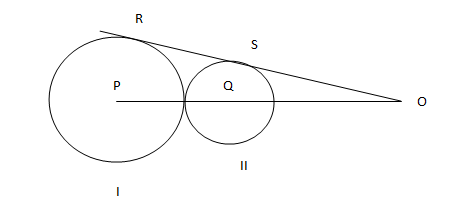# CAT 2004 Question Paper

Instructions

Directions for the following three questions: Answer the questions on the basis of the information given belowIn the adjoining figure I and II are circles with centres P and Q respectively, The two circles touch each other and have common tangent that touches them at points R and S respectively. This common tangent meets the line joining P and Q at O. The diameters of I and II are in the ratio 4: 3. It is also known that the length of PO is 28 cm.

Question 1

Question 2

Question 3

# The length of SO is[CAT 2004]

Instructions

Directions for the following two questions:

Answer the questions on the basis of the information given below.

$$f_1(x) = x$$ if $$0 \leq x \leq 1$$ $$f_1(x) = 1$$ if x >= 1 $$f_1(x) = 0$$ otherwise

$$f_2(x) = f_1(-x)$$ for all x

$$f_3(x) = -f_2(x)$$ for all x

$$f_4(x) = f_3(-x)$$ for all x

Question 4

Question 5

# Which of the following is necessarily true?

Instructions

Directions for the following two questions: Answer the questions on the basis of the information given below.

In an examination, there are 100 questions divided into three groups A, B and C such that each group contains at least one question. Each question in group A carries 1 mark, each question in group B carries 2 marks and each question in group C carries 3 marks. It is known that the questions in group A together carry at least 60% of the total marks.

Question 6

Question 7

# If group C contains 8 questions and group B carries at least 20% of the total marks, which of the following best describes the number of questions in group B?

Instructions

For the following questions answer them individually

Question 8

Question 9

Question 10

OR